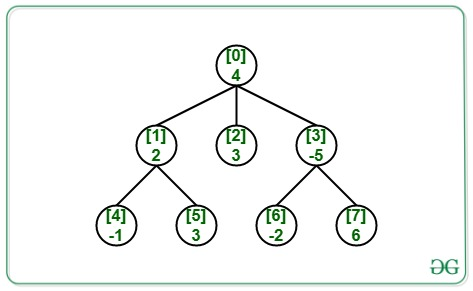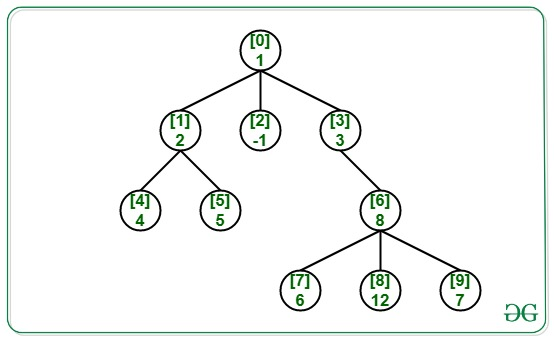Related Articles

# Maximum absolute difference between any two level sum in a N-ary Tree

• Last Updated : 21 Jun, 2021

Given an N-ary Tree having N nodes with positive and negative values and (N – 1) edges, the task is to find the maximum absolute difference of level sum in it.

Examples:

Input: N = 8, Edges[] = {{0, 1}, {0, 2}, {0, 3}, {1, 4}, {1, 5}, {3, 6}, {6, 7}}, Value[] = {4,2, 3, -5,-1, 3, -2, 6}, Below is the graph:Output: 6
Explanation:
Sum of all nodes of 0th level is 4.
Sum of all nodes of 1st level is 0.
Sum of all nodes of 2nd level is 6.
Hence, maximum absolute difference of level sum = (6 – 0) = 6.

Input: N = 10, Edges[] = {{0, 1}, {0, 2}, {0, 3}, {1, 4}, {1, 5}, {3, 6}, {6, 7}, {6, 8}, {6, 9}}, Value[] = {1, 2, -1, 3, 4, 5, 8, 6, 12, 7}, Below is the graph:Output: 24

Approach: To find the maximum absolute difference of level sum, first find the maximum level sum and minimum level sum because the absolute difference of maximum level sum and minimum level sum always gives us maximum absolute difference i.e.,

Maximum absolute difference = abs(Maximum level sum – Minimum level sum)

Below are the steps:

1. Perform the BFS Traversal on the give N-ary tree.
2. While doing the BFS traversal, process nodes of different levels separately.
3. For every level being processed, compute the sum of nodes in the level and keep track of maximum and minimum level sum.
4. After the above traversal, find the absolute difference of maximum and minimum level sum.

Below is the implementation of the above approach:

## C++

 `// C++ program for the above approach` `#include ``using` `namespace` `std;` `// Function to find the maximum``// absolute difference of level sum``void` `maxAbsDiffLevelSum(``int` `N, ``int` `M,``                        ``vector<``int``> cost,``                        ``int` `Edges[])``{``    ``// Create the adjacency list``    ``vector<``int``> adj[N];` `    ``for` `(``int` `i = 0; i < M; i++) {``        ``int` `u = Edges[i];``        ``int` `v = Edges[i];``        ``adj[u].push_back(v);``    ``}` `    ``// Initialize value of maximum``    ``// and minimum level sum``    ``int` `maxSum = cost, minSum = cost;` `    ``// Do Level order traversal keeping``    ``// track of nodes at every level``    ``queue<``int``> q;``    ``q.push(0);` `    ``while` `(!q.empty()) {` `        ``// Get the size of queue when``        ``// the level order traversal``        ``// for one level finishes``        ``int` `count = q.size();` `        ``int` `sum = 0;` `        ``// Iterate for all the nodes``        ``// in the queue currently``        ``while` `(count--) {` `            ``// Dequeue an node from queue``            ``int` `temp = q.front();``            ``q.pop();` `            ``sum = sum + cost[temp];` `            ``// Enqueue the children of``            ``// dequeued node``            ``for` `(``int` `i = 0;``                 ``i < adj[temp].size(); i++) {` `                ``q.push(adj[temp][i]);``            ``}``        ``}` `        ``// Update the maximum level``        ``// sum value``        ``maxSum = max(sum, maxSum);` `        ``// Update the minimum level``        ``// sum value``        ``minSum = min(sum, minSum);``    ``}` `    ``// Return the result``    ``cout << ``abs``(maxSum - minSum);``}` `// Driver Code``int` `main()``{``    ``// Number of nodes and edges``    ``int` `N = 10, M = 9;` `    ``// Edges of the N-ary tree``    ``int` `Edges[] = { { 0, 1 }, { 0, 2 },``                       ``{ 0, 3 }, { 1, 4 },``                       ``{ 1, 5 }, { 3, 6 },``                       ``{ 6, 7 }, { 6, 8 },``                       ``{ 6, 9 } };` `    ``// Given cost``    ``vector<``int``> cost = { 1, 2, -1, 3, 4,``                         ``5, 8, 6, 12, 7 };` `    ``// Function Call``    ``maxAbsDiffLevelSum(N, M, cost, Edges);` `    ``return` `0;``}`

## Java

 `// Java program for the above approach``import` `java.util.*;` `class` `GFG{` `// Function to find the maximum``// absolute difference of level sum``static` `void` `maxAbsDiffLevelSum(``int` `N, ``int` `M,``                               ``int` `[]cost,``                               ``int` `Edges[][])``{``    ` `    ``// Create the adjacency list``    ``@SuppressWarnings``(``"unchecked"``)``    ``Vector []adj = ``new` `Vector[N];``    ``for``(``int` `i = ``0``; i < adj.length; i++)``        ``adj[i] = ``new` `Vector();``        ` `    ``for``(``int` `i = ``0``; i < M; i++)``    ``{``        ``int` `u = Edges[i][``0``];``        ``int` `v = Edges[i][``1``];``        ``adj[u].add(v);``    ``}` `    ``// Initialize value of maximum``    ``// and minimum level sum``    ``int` `maxSum = cost[``0``], minSum = cost[``0``];` `    ``// Do Level order traversal keeping``    ``// track of nodes at every level``    ``Queue q = ``new` `LinkedList();``    ``q.add(``0``);` `    ``while` `(!q.isEmpty())``    ``{``        ` `        ``// Get the size of queue when``        ``// the level order traversal``        ``// for one level finishes``        ``int` `count = q.size();` `        ``int` `sum = ``0``;` `        ``// Iterate for all the nodes``        ``// in the queue currently``        ``while` `(count-- > ``0``)``        ``{` `            ``// Dequeue an node from queue``            ``int` `temp = q.peek();``            ``q.remove();` `            ``sum = sum + cost[temp];` `            ``// Enqueue the children of``            ``// dequeued node``            ``for``(``int` `i = ``0``;``                    ``i < adj[temp].size();``                    ``i++)``            ``{``                ``q.add(adj[temp].get(i));``            ``}``        ``}` `        ``// Update the maximum level``        ``// sum value``        ``maxSum = Math.max(sum, maxSum);` `        ``// Update the minimum level``        ``// sum value``        ``minSum = Math.min(sum, minSum);``    ``}` `    ``// Return the result``    ``System.out.print(Math.abs(maxSum - minSum));``}` `// Driver Code``public` `static` `void` `main(String[] args)``{``    ` `    ``// Number of nodes and edges``    ``int` `N = ``10``, M = ``9``;` `    ``// Edges of the N-ary tree``    ``int` `Edges[][] = { { ``0``, ``1` `}, { ``0``, ``2` `},``                      ``{ ``0``, ``3` `}, { ``1``, ``4` `},``                      ``{ ``1``, ``5` `}, { ``3``, ``6` `},``                      ``{ ``6``, ``7` `}, { ``6``, ``8` `},``                      ``{ ``6``, ``9` `} };` `    ``// Given cost``    ``int` `[]cost = { ``1``, ``2``, -``1``, ``3``, ``4``,``                   ``5``, ``8``, ``6``, ``12``, ``7` `};` `    ``// Function call``    ``maxAbsDiffLevelSum(N, M, cost, Edges);``}``}` `// This code is contributed by Amit Katiyar`

## Python3

 `# Python3 program for the above approach``from` `collections ``import` `deque` `# Function to find the maximum``# absolute difference of level sum``def` `maxAbsDiffLevelSum(N, M, cost, Edges):` `    ``# Create the adjacency list``    ``adj ``=` `[[] ``for` `i ``in` `range``(N)]` `    ``for` `i ``in` `range``(M):``        ``u ``=` `Edges[i][``0``]``        ``v ``=` `Edges[i][``1``]``        ``adj[u].append(v)` `    ``# Initialize value of maximum``    ``# and minimum level sum``    ``maxSum ``=` `cost[``0``]``    ``minSum ``=` `cost[``0``]` `    ``# Do Level order traversal keeping``    ``# track of nodes at every level``    ``q ``=` `deque()``    ``q.append(``0``)` `    ``while` `len``(q) > ``0``:` `        ``# Get the size of queue when``        ``# the level order traversal``        ``# for one level finishes``        ``count ``=` `len``(q)` `        ``sum` `=` `0` `        ``# Iterate for all the nodes``        ``# in the queue currently``        ``while` `(count):` `            ``# Dequeue an node from queue``            ``temp ``=` `q.popleft()``            ``# q.pop()` `            ``sum` `=` `sum` `+` `cost[temp]` `            ``# Enqueue the children of``            ``# dequeued node``            ``for` `i ``in` `adj[temp]:``                ``q.append(i)``                ` `            ``count ``-``=` `1` `        ``# Update the maximum level``        ``# sum value``        ``maxSum ``=` `max``(``sum``, maxSum)` `        ``# Update the minimum level``        ``# sum value``        ``minSum ``=` `min``(``sum``, minSum)` `    ``# Return the result``    ``print``(``abs``(maxSum ``-` `minSum))` `# Driver Code``if` `__name__ ``=``=` `'__main__'``:``    ` `    ``# Number of nodes and edges``    ``N ``=` `10``    ``M ``=` `9` `    ``# Edges of the N-ary tree``    ``Edges ``=` `[ [ ``0``, ``1` `], [ ``0``, ``2` `],``              ``[ ``0``, ``3` `], [ ``1``, ``4` `],``              ``[ ``1``, ``5` `], [ ``3``, ``6` `],``              ``[ ``6``, ``7` `], [ ``6``, ``8` `],``              ``[ ``6``, ``9` `] ]` `    ``# Given cost``    ``cost ``=` `[ ``1``, ``2``, ``-``1``, ``3``, ``4``,``             ``5``, ``8``, ``6``, ``12``, ``7` `]` `    ``# Function call``    ``maxAbsDiffLevelSum(N, M, cost, Edges)` `# This code is contributed by mohit kumar 29`

## C#

 `// C# program for``// the above approach``using` `System;``using` `System.Collections.Generic;``class` `GFG{` `// Function to find the maximum``// absolute difference of level sum``static` `void` `maxAbsDiffLevelSum(``int` `N, ``int` `M,``                               ``int` `[]cost,``                               ``int` `[,]Edges)``{``  ``// Create the adjacency list``  ``List<``int``> []adj = ``new` `List<``int``>[N];``  ``for``(``int` `i = 0; i < adj.Length; i++)``    ``adj[i] = ``new` `List<``int``>();` `  ``for``(``int` `i = 0; i < M; i++)``  ``{``    ``int` `u = Edges[i, 0];``    ``int` `v = Edges[i, 1];``    ``adj[u].Add(v);``  ``}` `  ``// Initialize value of maximum``  ``// and minimum level sum``  ``int` `maxSum = cost, minSum = cost;` `  ``// Do Level order traversal keeping``  ``// track of nodes at every level``  ``Queue<``int``> q = ``new` `Queue<``int``>();``  ``q.Enqueue(0);` `  ``while` `(q.Count!=0)``  ``{``    ``// Get the size of queue when``    ``// the level order traversal``    ``// for one level finishes``    ``int` `count = q.Count;` `    ``int` `sum = 0;` `    ``// Iterate for all the nodes``    ``// in the queue currently``    ``while` `(count-- > 0)``    ``{``      ``// Dequeue an node from queue``      ``int` `temp = q.Peek();``      ``q.Dequeue();` `      ``sum = sum + cost[temp];` `      ``// Enqueue the children of``      ``// dequeued node``      ``for``(``int` `i = 0; i < adj[temp].Count; i++)``      ``{``        ``q.Enqueue(adj[temp][i]);``      ``}``    ``}` `    ``// Update the maximum level``    ``// sum value``    ``maxSum = Math.Max(sum, maxSum);` `    ``// Update the minimum level``    ``// sum value``    ``minSum = Math.Min(sum, minSum);``  ``}` `  ``// Return the result``  ``Console.Write(Math.Abs(maxSum - minSum));``}` `// Driver Code``public` `static` `void` `Main(String[] args)``{   ``  ``// Number of nodes and edges``  ``int` `N = 10, M = 9;` `  ``// Edges of the N-ary tree``  ``int` `[,]Edges = {{0, 1}, {0, 2},``                  ``{0, 3}, {1, 4},``                  ``{1, 5}, {3, 6},``                  ``{6, 7}, {6, 8},``                  ``{6, 9}};` `  ``// Given cost``  ``int` `[]cost = {1, 2, -1, 3, 4,``                ``5, 8, 6, 12, 7};` `  ``// Function call``  ``maxAbsDiffLevelSum(N, M, cost, Edges);``}``}` `// This code is contributed by 29AjayKumar`

## Javascript

 ``
Output:
`24`

Time Complexity: O(N)
Auxiliary Space: O(N)

Attention reader! Don’t stop learning now. Get hold of all the important DSA concepts with the DSA Self Paced Course at a student-friendly price and become industry ready.  To complete your preparation from learning a language to DS Algo and many more,  please refer Complete Interview Preparation Course.

In case you wish to attend live classes with experts, please refer DSA Live Classes for Working Professionals and Competitive Programming Live for Students.

My Personal Notes arrow_drop_up